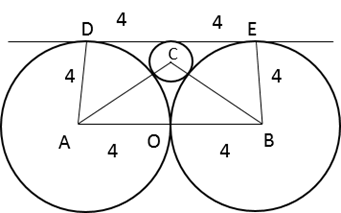# CAT 2019 Question Paper | Quants Slot 2

###### CAT Previous Year Paper | CAT Quants Questions | Question 7

Geometry is one topic that falls under the Arithmetic part of the Quantitative Ability section of the CAT exam and is the second most tested topic in this section. Find more details about the importance of this topic by visiting 2IIM's CAT Blueprint . Practising for a topic this important is extremely crucial and the best way to go about it is to solve questions asked in CAT previous year paper. Be thorough in your online CAT Preparation by taking out the time to religiously practice these questions. Start with this question on Geometry from CAT 2019 Question Paper Slot 2 right now.

Question 7 : Two circles, each of radius 4 cm, touch externally. Each of these two circles is touched externally by a third circle. If these three circles have a common tangent, then the radius of the third circle, in cm, is

1. $$frac{π}{3}$ 2. 1 3. $\frac{1}{\sqrt{2}}$ 4. $\sqrt{2}$ ## Best CAT Online Coaching Try upto 40 hours for free Learn from the best! #### 2IIM : Best Online CAT Coaching. ### Video Explanation ## Best CAT Coaching in Chennai #### CAT Coaching in Chennai - CAT 2022Limited Seats Available - Register Now! ### Explanatory AnswerFor three circles to have a common tangent, the third circle must lie in between the two circles. Let the radius of the third circle be r cms Now, Connect the centres of the circles with one another and with the intersection points. Let them be A,B,C,D,E respectively. Now, from observing the constructed diagram, we find AB = 8cms AC =$4 + r) cms
BC = (4 + r) cms
OC = (4 - r) cms
Now, consider the right triangle OBC,
Applying Pythagoras theorem,
(4+r)2 = 42 + (4 - r)2
16 + r2 + 8r = 16 + 16 + r2 - 8r
16r = 16
r = 1 cms

The question is "Two circles, each of radius 4 cm, touch externally. Each of these two circles is touched externally by a third circle. If these three circles have a common tangent, then the radius of the third circle, in cm, is"

##### Hence, the answer is 1

Choice B is the correct answer.

###### CAT Coaching in ChennaiCAT 2023

Classroom Batches Starting Now! @Gopalapuram

###### Best CAT Coaching in Chennai Introductory offer of 5000/-

Attend a Demo Class

##### Where is 2IIM located?

2IIM Online CAT Coaching
A Fermat Education Initiative,
58/16, Indira Gandhi Street,
Kaveri Rangan Nagar, Saligramam, Chennai 600 093

##### How to reach 2IIM?

Mobile: (91) 99626 48484 / 94459 38484
WhatsApp: WhatsApp Now
Email: info@2iim.com